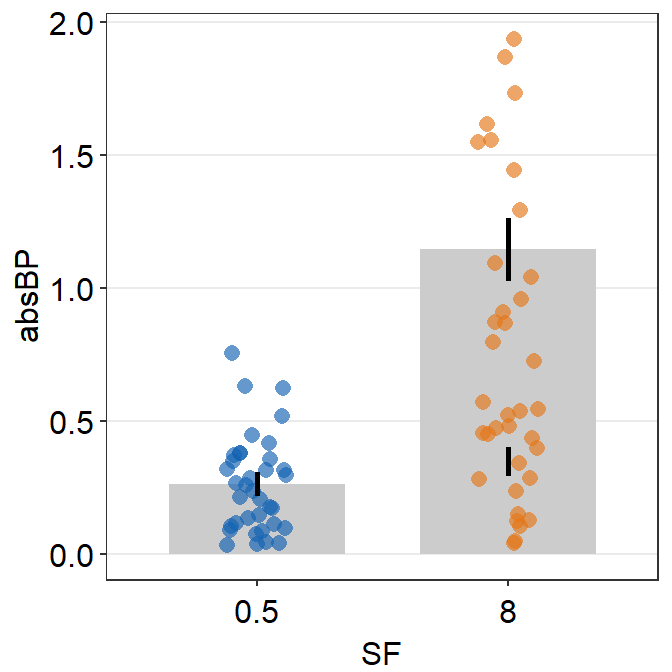# Chapter 4 Manual and Examples of smplot

This chapter is a manual for smplot; it includes numerous examples. It also includes tutorials about sm_bar(), sm_bland_altman() and sm_raincloud(), all of which are not mentioned in the preceding chapters. However, this chapter does not describe sm_effsize(), sm_power() and sm_common_axis(); these functions are described in Chapters 5-7.

• If you are not sure about any of the functions, please type ? before the function names, ex. ?sm_bar.

## 4.1 Installation of the Package

• The smplot package is NOT available on CRAN yet. So, you will need to download it directly from my github for now.
• To install it, please type in the R console:
install.packages('devtools')
devtools::install_github('smin95/smplot')
• To use the package, load it:
library(smplot)

### What is smplot?

• smplot is a package that provides functions that visually improve graphs produced from ggplot2.
• So it does not work with plots made from base R.
• It was first developed in May 2021.
• It is free and open source (https://github.com/smin95/smplot).

## 4.2 smplot’s Color Palette and Graph Themes

### smplot’s color palette

• Its color palette can be accessed via two functions: sm_color() and sm_palette().
• sm_color() accepts the character string of the color name.
• sm_palette() accepts the number of colors (up to 20) and returns the hex codes accordingly.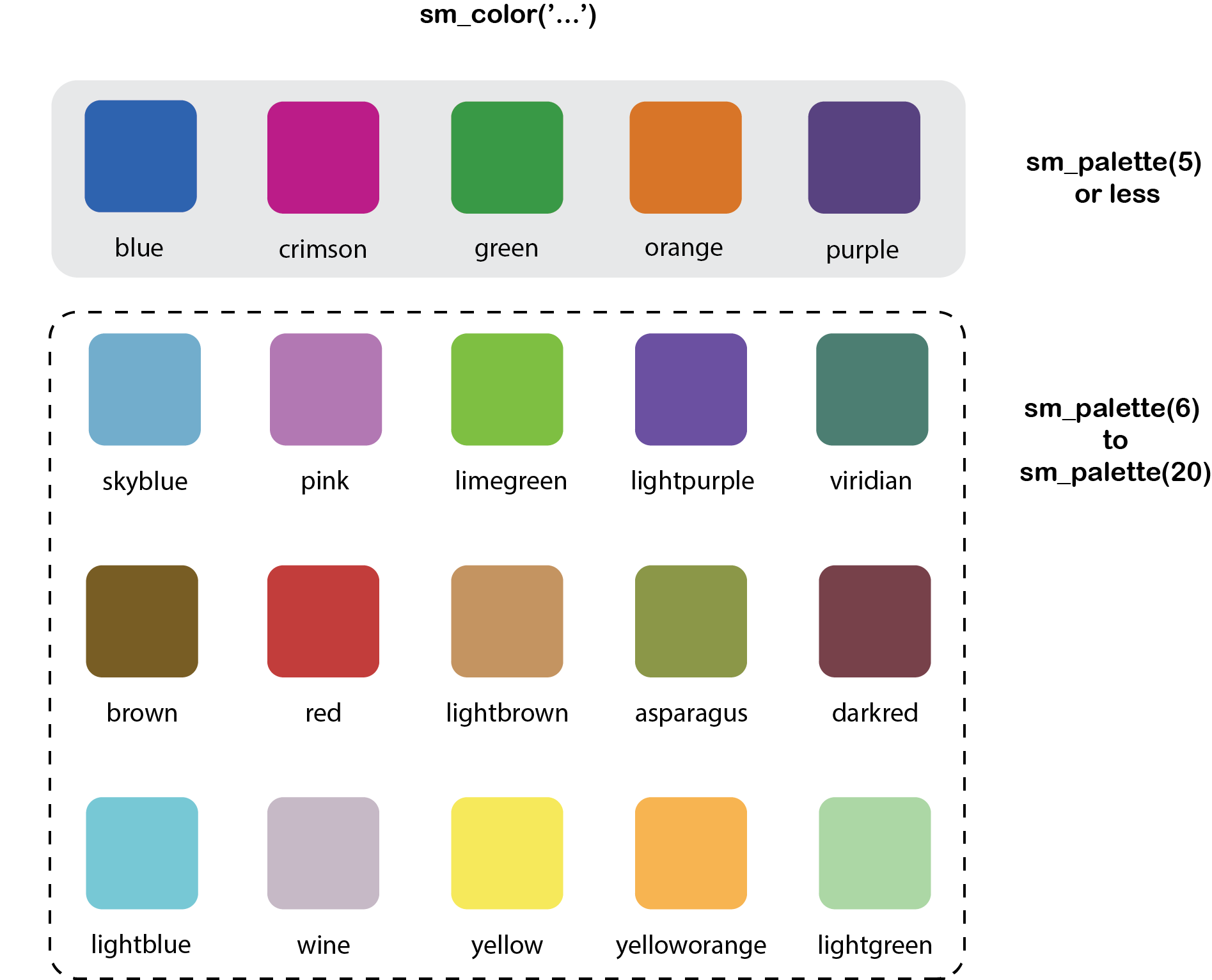Figure 4.1: smplot’s color palette

• For example, if you want blue and red, just type the input like this:
sm_color('blue','red')
##  "#1262b3" "#cc3d3d"
• But, do not form a single vector that contains two characters. If so, sm_color() will only return the first color.
sm_color(c('blue','red'))
## Warning in if (color == "blue") return("#1262b3"): the condition has length
## > 1 and only the first element will be used
##  "#1262b3"
• If you need 5 colors, you can use sm_palette().
sm_palette(5)
##  "#cc1489" "#1262b3" "#5b4080" "#e57717" "#0f993d"

### smplot’s graph themes

• There are several its graph themes. The text positions and the font are all similar.
• sm_corr_theme() and sm_hvgrid() are equivalent. They have major horizontal and vertical grids.
• This theme is useful for correlation, so I created a duplicate function sm_corr_theme().
• sm_bar_theme() and sm_hgrid() are equivalent. They have major horizontal grids.
• This theme is useful for a bar graph, so I created a duplicate function sm_bar_theme().
• sm_minimal has no major grid. This is useful when a graph has a lot of annotation, such as texts and arrows.
• sm_slope_theme() is a theme for a slope chart. It removes everything except the y-axis.
• All of these functions, except for sm_slope_theme(), have two arguments: borders and legends.
• For some of these functions, the defaults are set to borders = TRUE and legends = TRUE.
• You can check the defaults by typing ? in front of the function. Ex: ?sm_corr_theme
• There is no option for borders in sm_slope_theme().
• p1 has the default theme of ggplot2.
library(tidyverse)
p1 <- ggplot(data = mpg, mapping = aes(x = displ, y = hwy, color = class)) +
geom_point(size = 2)
p1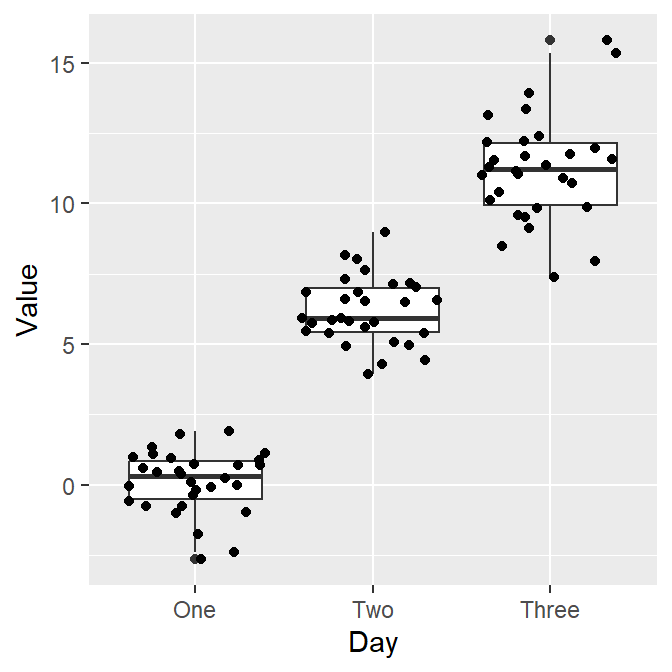• Now we can change the theme using sm_corr_theme().
p1 + sm_corr_theme()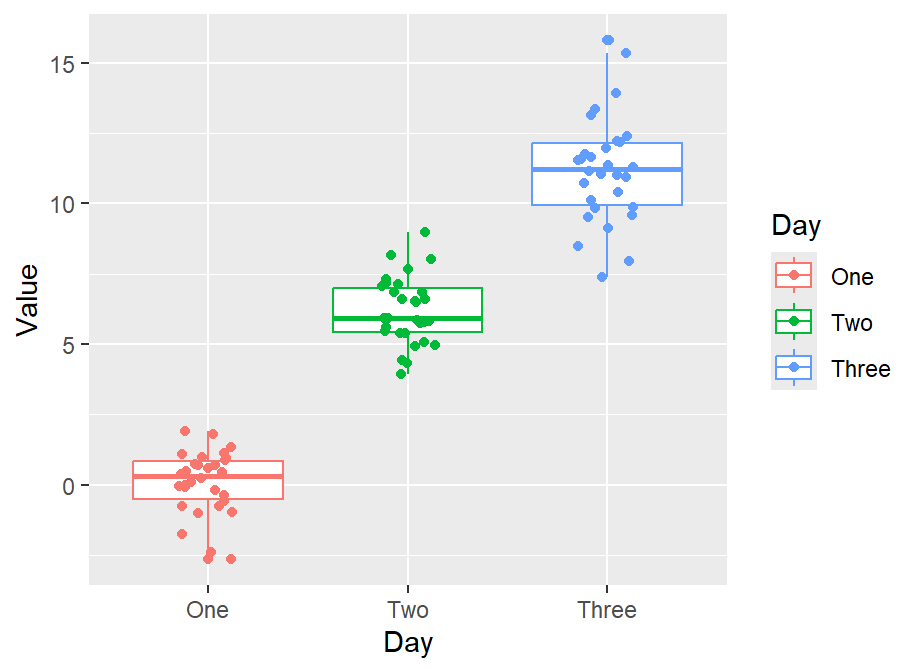• We can also remove borders and legends by setting them as FALSE.
p2 <- p1 + sm_corr_theme(borders = FALSE, legends = FALSE)
p2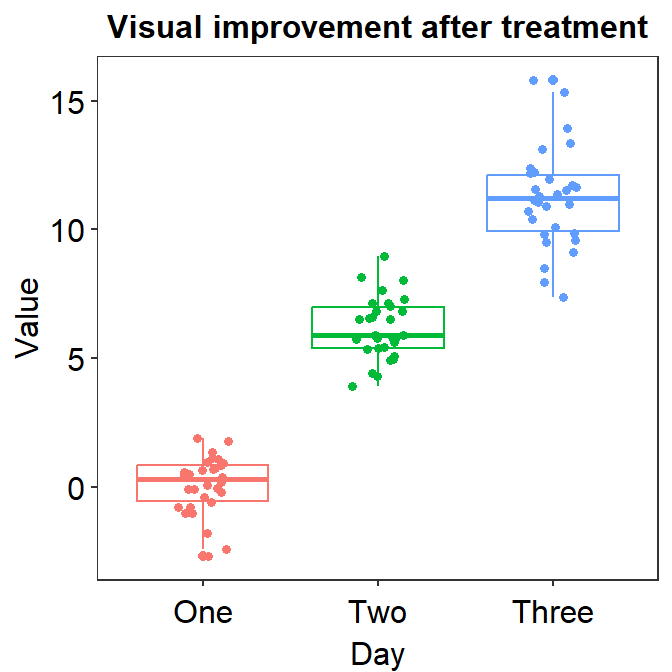• You can also apply smplot’s colors by using scale_color_manual().
p2 + scale_color_manual(values = sm_palette(7))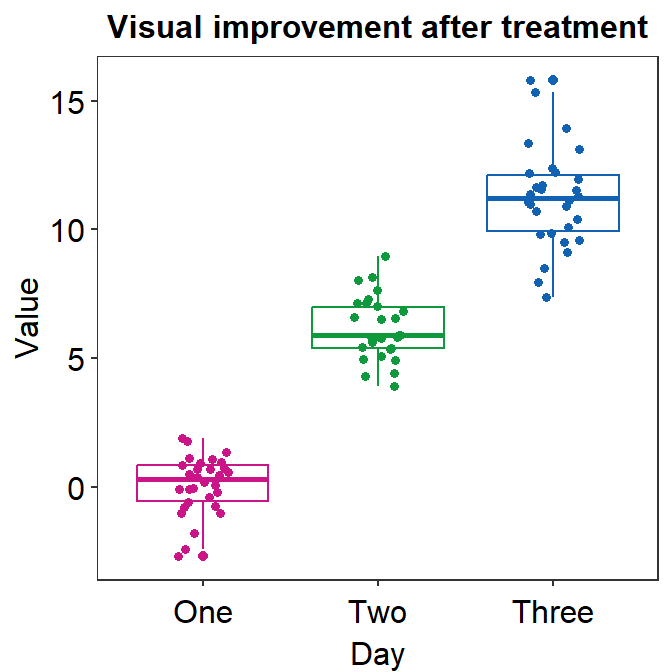## 4.3 Correlation Plot

• sm_corr_theme() and sm_statCorr() can be used as a pair when plotting a correlation.

• This is the plot using the default theme of ggplot2.

p1 <- ggplot(data = mtcars, mapping = aes(x = drat, y = mpg)) +
geom_point(shape = 21, fill = sm_color('green'), color = 'white', size = 3)
p1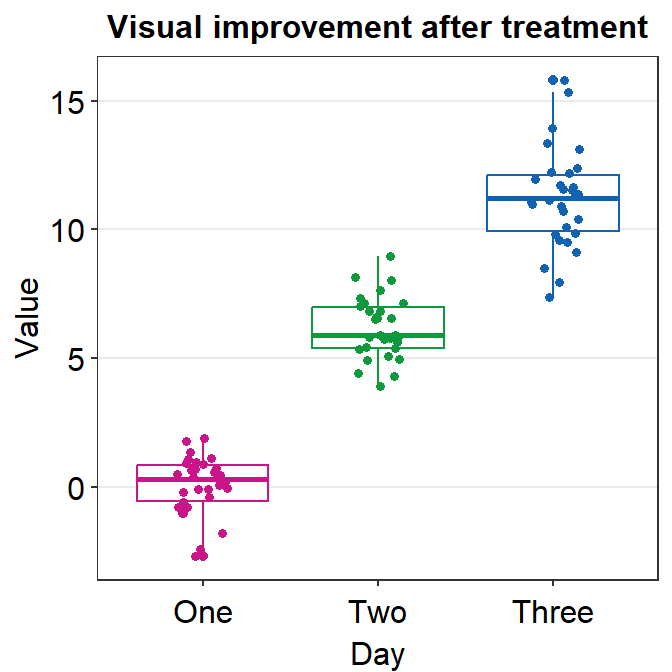• The next plot uses sm_corr_theme() to apply the smplot’s theme and sm_statCorr() to print linear regression slope and statistical results from a paired correlation test (Pearson’s).
• Important: sm_statCorr() recognizes the data for the y- and x-axes from the mapping = aes() in ggplot() function.
• There is no mapping argument in sm_statCorr().
p1 + sm_corr_theme() +
sm_statCorr(color = sm_color('green'))• You can also change the line_type to 'solid' in sm_statCorr(), and change the location of the printed texts by using label_x and label_y arguments.
• You can also change the font size of the printed texts by setting text_size to a larger numerical value.
p1 + sm_corr_theme() +
sm_statCorr(color = sm_color('green'),
line_type = 'solid',
label_x = 3.7,
label_y = 11,
text_size = 5)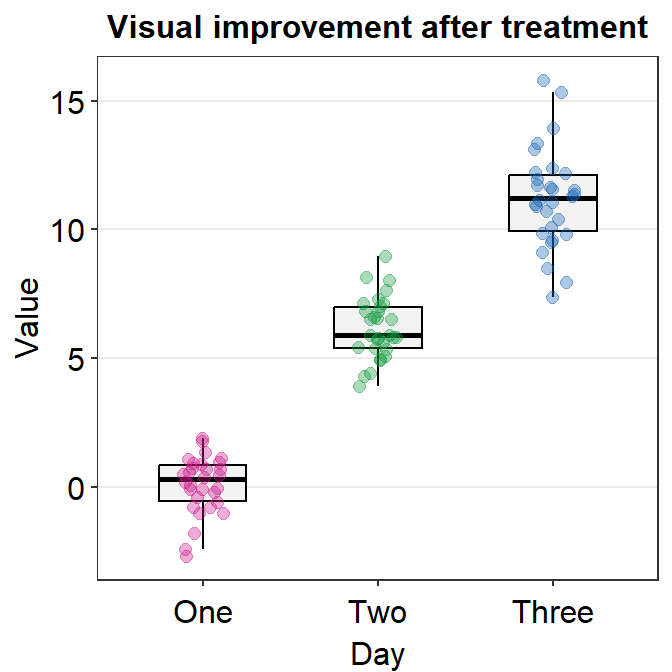### 4.3.1 Data frame for a correlation plot

• Column 1 has to be the data for x-axis.

• Column 2 has to be the data for y-axis.

• This structure of the data frame is slightly different from that is typically used in ggplot2 and smplot functions (ex. sm_boxplot(), sm_bar(), sm_violin() and sm_raincloud()).

• Correlation plot and a bar plot requires a different data frame structure.

# Example
set.seed(11) # generate random data
method1 = c(rnorm(19,0,1),2.5)
method2 = c(rnorm(19,0,1),2.5)
Subject <- rep(paste0('S',seq(1:20)), 2)
Data <- data.frame(Value = matrix(c(method1,method2),ncol=1))
Method <- rep(c('Method 1', 'Method 2'), each = length(method1))
df_general <- cbind(Subject, Data, Method) # used for sm_bar(), sm_boxplot(), sm_violin(), etc

df_corr <- data.frame(first = method1, second = method2) # used for correlation
• We have created two data frames: df_general and df_corr. Let’s take a look at their structures.
head(df_general)
##   Subject       Value   Method
## 1      S1 -0.59103110 Method 1
## 2      S2  0.02659437 Method 1
## 3      S3 -1.51655310 Method 1
## 4      S4 -1.36265335 Method 1
## 5      S5  1.17848916 Method 1
## 6      S6 -0.93415132 Method 1
• Notice that df_general has three columns. The first column is subject, second column is data (i.e., Value) and third column is measurement group.
head(df_corr)
##         first      second
## 1 -0.59103110 -0.65571812
## 2  0.02659437 -0.68251762
## 3 -1.51655310 -0.01585819
## 4 -1.36265335 -0.44260479
## 5  1.17848916  0.35255750
## 6 -0.93415132  0.07317058
• Notice that df_corr has two columns, each of which represents a measurement group.
# correlation plot using data frame 'df_corr'
ggplot(data = df_corr, mapping = aes(x = first, y = second)) +
geom_point(shape = 21, fill = sm_color('crimson'), color = 'white',
size = 3) + sm_corr_theme(borders = FALSE) +
scale_y_continuous(limits = c(-2.5,2.5)) +
scale_x_continuous(limits = c(-2.5,2.5)) +
sm_statCorr(color = sm_color('crimson'), corr_method = 'pearson',
label_x = -2.2, label_y = 2.3) +
ggtitle('Correlation plot') +
xlab('Method 1') + ylab('Method 2')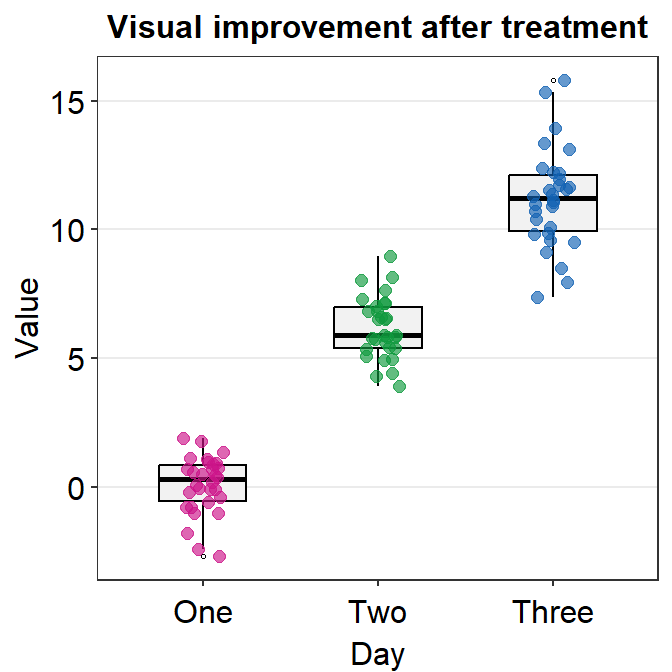# bar graph using data frame 'df_general'
ggplot(data = df_general, mapping = aes(x = Method, y = Value, fill = Method)) +
sm_bar(shape = 21, color = 'white', bar_fill_color = 'gray80') +
scale_fill_manual(values = sm_color('crimson','green'))### 4.3.2 Correlation plot with both regression and reference lines

• You can also add a reference line (slope = 1) in a correlation plot.
• This can be done with geom_abline(). In this example, the reference line’s slope is set to 1 and it has a dashed line style.
# correlation plot using data frame 'df_corr'
ggplot(data = df_corr, mapping = aes(x = first, y = second)) +
geom_point(shape = 21, fill = sm_color('crimson'), color = 'white',
size = 3) + sm_corr_theme(borders = FALSE) +
geom_abline(slope = 1, linetype = 'dashed') +
scale_y_continuous(limits = c(-2.8,2.8),  expand = c(0,0)) +
scale_x_continuous(limits = c(-2.8,2.8), expand = c(0,0)) +
sm_statCorr(color = sm_color('crimson'), corr_method = 'pearson',
label_x = -2.2, label_y = 2.3) +
ggtitle('Correlation plot') +
xlab('Method 1') + ylab('Method 2')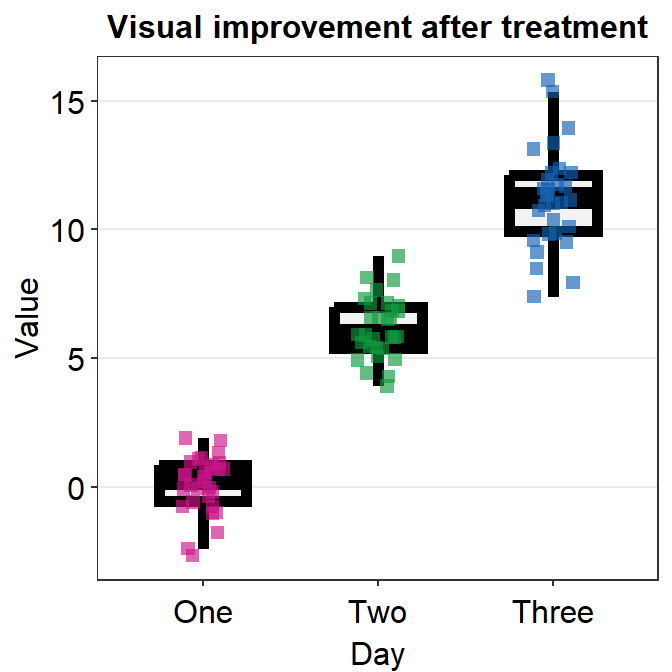## 4.4 Boxplot - sm_boxplot()

• sm_boxplot() generates a boxplot and individual points at the same time.
• It automatically uses sm_hgrid() as its default theme.
• It also has arguments borders and legends, both of which have been set to FALSE as defaults.
• First, let’s generate some random data.
set.seed(1) # generate random data
day1 = rnorm(16,0,1)
day2 = rnorm(16,5,1)
Subject <- rep(paste0('S',seq(1:16)), 2)
Data <- data.frame(Value = matrix(c(day1,day2),ncol=1))
Day <- rep(c('Day 1', 'Day 2'), each = length(day1))
df <- cbind(Subject, Data, Day)
• Now, let’s make a boxplot using sm_boxplot().
# a boxplot with the random data, all black points
ggplot(data = df, mapping = aes(x = Day, y = Value)) +
sm_boxplot(fill = 'black')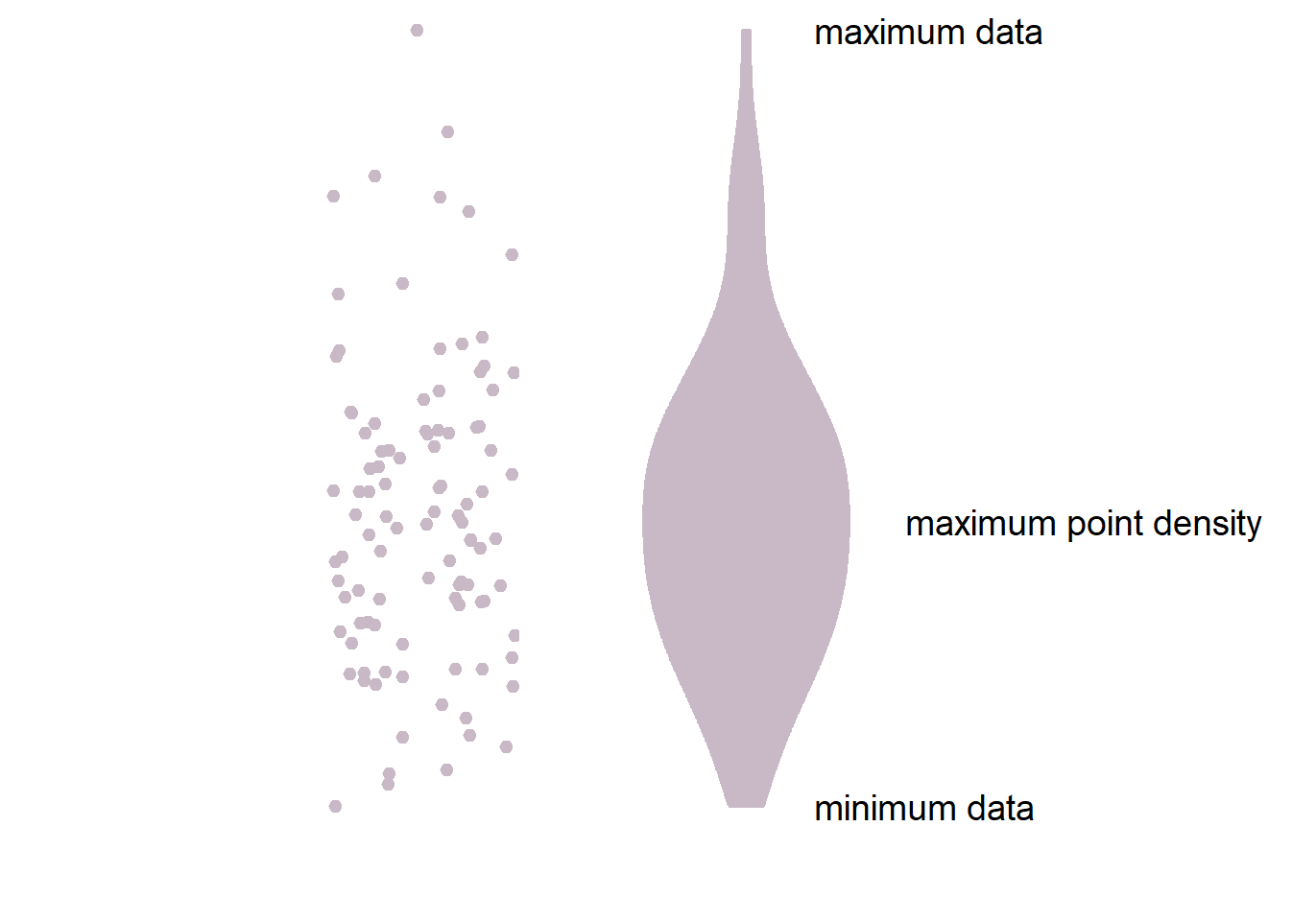• Now let’s apply different color for each Day.
# a boxplot with different colored points
ggplot(data = df, mapping = aes(x = Day, y = Value, fill = Day)) +
sm_boxplot(shape = 21, color = 'white') +
scale_fill_manual(values = sm_color('blue','orange'))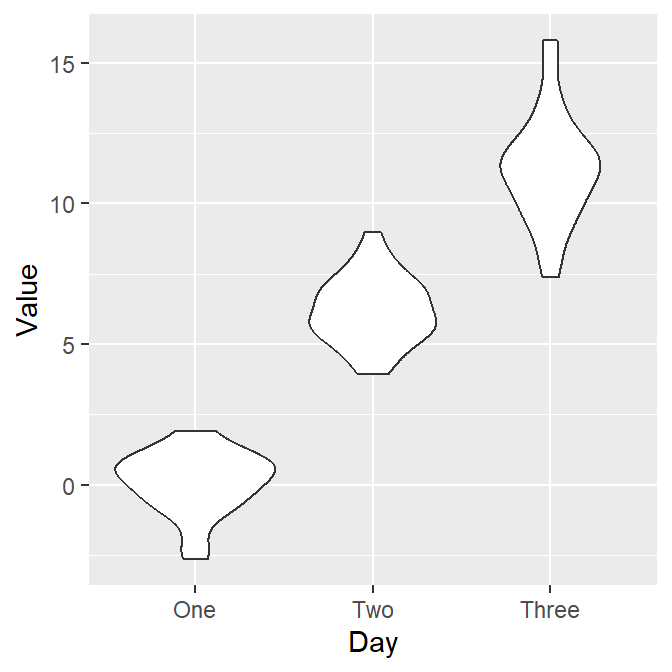• You can change the shape of the boxplot by setting notch = TRUE. You can also change the size of the individual points using point_size argument.
• A notched boxplot shows the confidence interval around the median (+/- 1.58 * interquartile range / sqrt(n)).
• The notches are used for group comparison.
• If the notch of each box does not overlap, there is a strong likelihood that the medians are significantly different between groups.
ggplot(data = df, mapping = aes(x = Day, y = Value, fill = Day)) +
sm_boxplot(shape = 21, point_size = 4, notch = 'TRUE', alpha = 0.5) +
scale_fill_manual(values = sm_color('blue','orange'))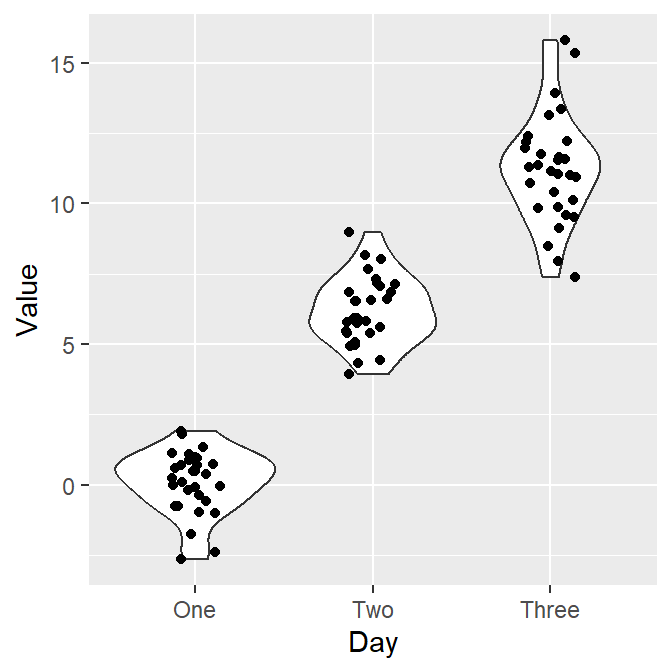### 4.4.1 Plotting individual points with unique colors

• One can also use sm_boxplot() to plot individual points with unique colors.
• However, sm_boxplot() cannot print distinct box colors across distinct x levels (i.e., in this example, all boxes are grey). This is because I think that it is not a good practice to print different colors of boxes when each point has unique color as well.
ggplot(data = df, mapping = aes(x = Day, y = Value, fill = Subject)) +
sm_boxplot(shape = 21, color = 'white') +
scale_fill_manual(values = sm_palette(16))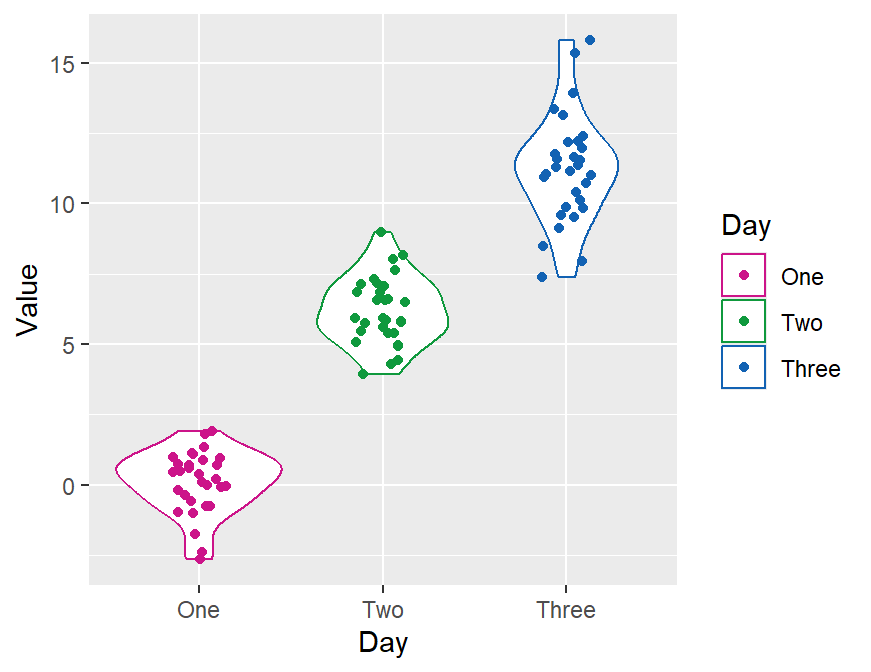## 4.5 Violin Plot - sm_violin()

• sm_violin() plots a violin plot, individual points and lines that indicate means and +/- 1 standard deviation at the same time.
• It is very similar to sm_boxplot() except there is no option for notch = TRUE in sm_violin().
• Also sm_violin() uses both color (for the lines of mean and SD) and fill (for the colors of the points) arguments.
• The default border color of the points is white.
• sm_violin() automatically uses sm_hgrid() as its default theme.
• It also has arguments borders and legends, both of which have been set to FALSE as defaults.
# a violin plot with the random data, all black points and lines
ggplot(data = df, mapping = aes(x = Day, y = Value)) +
sm_violin(fill = 'black')# a violin plot with different colored points and lines
ggplot(data = df, mapping = aes(x = Day, y = Value, color = Day)) +
sm_violin() +
scale_color_manual(values = sm_color('blue','orange'))### 4.5.1 Plotting individual points with unique colors

• One can also use sm_violin() to plot individual points with unique colors.
• The x-level has to be grouped in the aesthetics (ex. group = Day).
• But sm_violin() cannot print distinct violin colors across distinct x levels (i.e., in this example, all violins are grey). This is because it is aesthetically distracting to assign different colors of the violin across distinct x-levels.
ggplot(data = df, mapping = aes(x = Day, y = Value, fill = Subject,
group = Day)) +
sm_violin(shape = 21, color = 'white', point_alpha = 0.6) +
scale_fill_manual(values = sm_palette(16))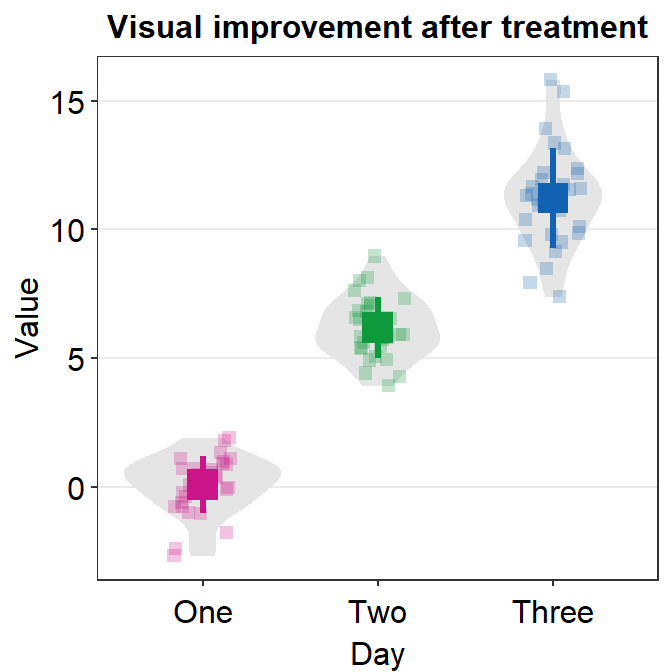ggplot(data = df, mapping = aes(x = Day, y = Value, fill = Subject,
group = Day, color = Day)) +
sm_violin(shape = 21, color = 'white', point_alpha = 0.6) +
scale_fill_manual(values = sm_palette(16)) +
scale_color_manual(values = sm_color('blue', 'orange'))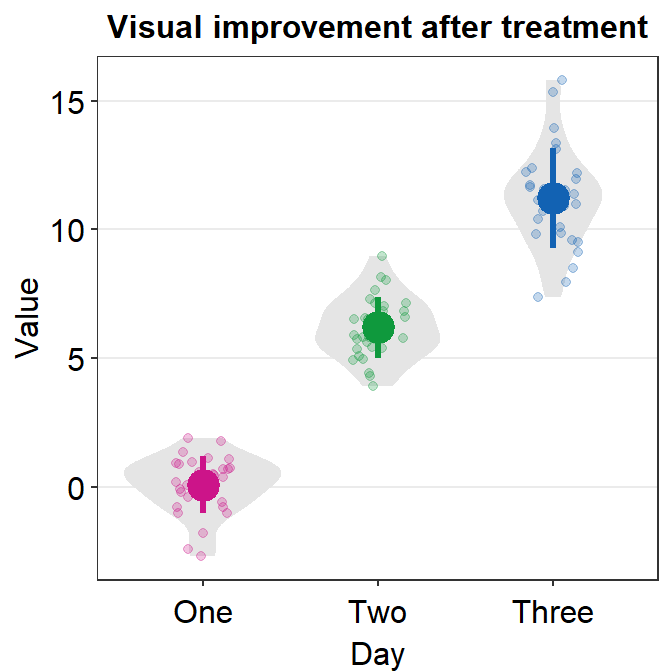## 4.6 Bar Plot - sm_bar()

• sm_bar() automatically uses sm_bar_theme() / sm_hgrid().
• It also has arguments borders and legends, both of which have been set to FALSE as defaults.
• Let’s use data (df) we generated before.
ggplot(data = df, mapping = aes(x = Day, y = Value, fill = Day)) +
sm_bar(shape = 21, color = 'white', bar_fill_color = 'gray80') +
scale_fill_manual(values = sm_color('blue','orange'))• In this case, the error bar represents standard error. If you prefer to show standard deviation, then you should set errorbar_type = 'sd' in sm_bar().
ggplot(data = df, mapping = aes(x = Day, y = Value, fill = Day)) +
sm_bar(shape = 21, color = 'white', bar_fill_color = 'gray80', errorbar_type = 'sd') +
scale_fill_manual(values = sm_color('blue','orange'))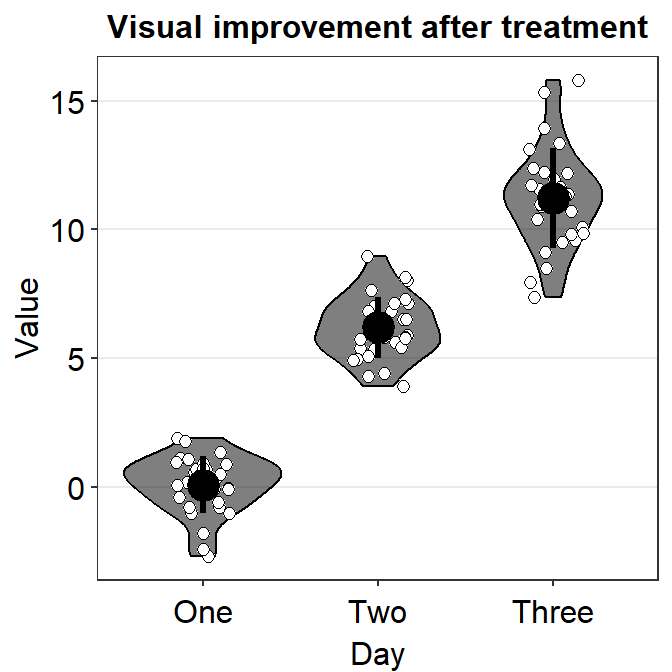• 95% confidence interval also be displayed with errorbar_type = 'ci'.
ggplot(data = df, mapping = aes(x = Day, y = Value, fill = Day)) +
sm_bar(shape = 21, color = 'white', bar_fill_color = 'gray80', errorbar_type = 'ci') +
scale_fill_manual(values = sm_color('blue','orange'))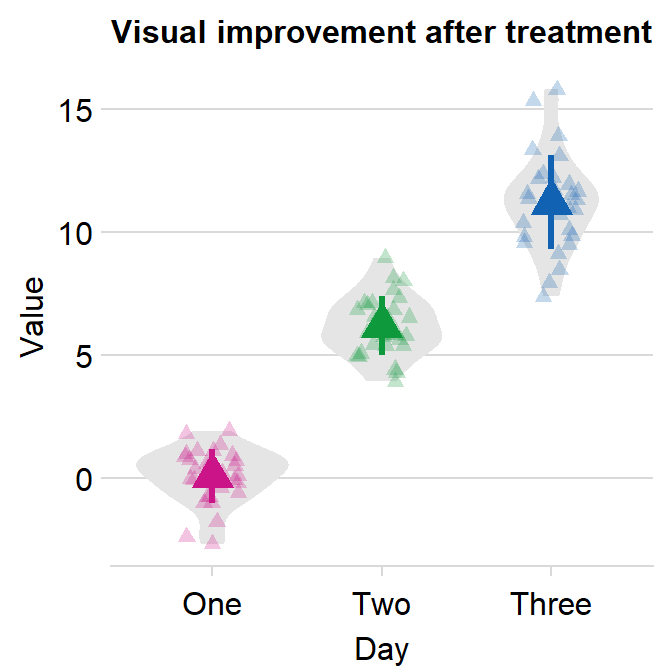### 4.6.1 Plotting individual points with unique colors

• One can also use sm_bar() to plot individual points with unique colors.
ggplot(data = df, mapping = aes(x = Day, y = Value, color = Subject)) +
sm_bar(bar_fill_color = 'gray80') +
scale_color_manual(values = sm_palette(16))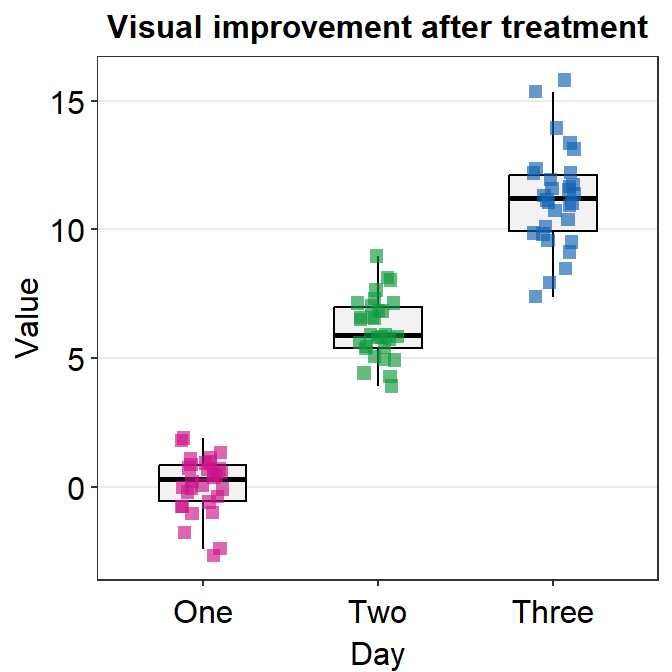• sm_bar() can also print distinct box colors across distinct x levels.
ggplot(data = df, mapping = aes(x = Day, y = Value, color = Subject,
fill = Day)) +
sm_bar() +
scale_color_manual(values = sm_palette(16)) +
scale_fill_manual(values = sm_color('yelloworange','skyblue'))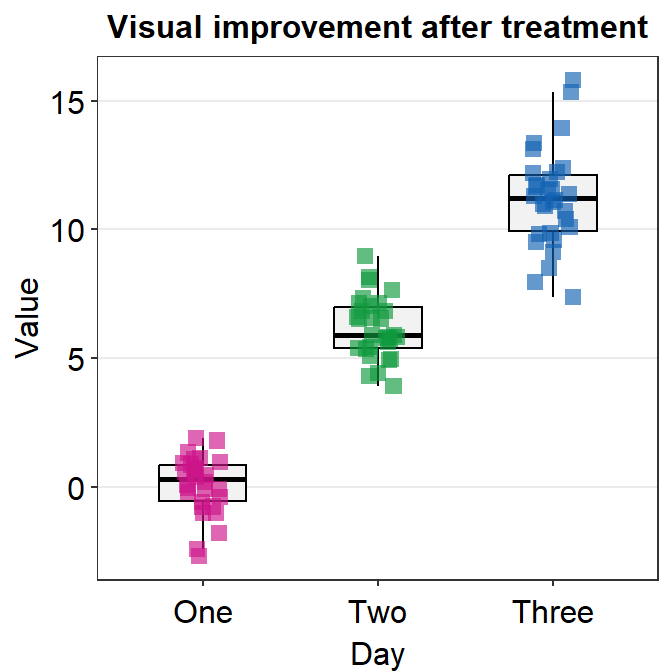## 4.7 Slope Chart - sm_slope()

• sm_slope() plots a slope chart.
• It also has the argument legends, which has been set to FALSE as the default.
• A slope chart is useful to describe changes between two different timepoints for each measurement (ex. a participant).
• It automatically uses sm_slope_theme().
• Let’s use df that we generated before.
• Important: To make this function work, the mapping within ggplot() has to have a certain structure.
• x- and y-axes have to be defined.
• A slope chart groups each observation (ex. Subject) across x-axis. This has to be specified in mapping as group =.
• The x-axis cannot be continuous. It has to be discrete. So, it should take the form of character or factor (ex. ‘One’, ‘Two’, ‘Three’). If x-axis only has number (i.e., double form, such as 1.02, 1.05, 1.5), then sm_slope() will produce an error.
• labels argument is required to use sm_slope(). This refers to the labels of the ticks in the x-axis. Ex. labels = c('Day 1', 'Day 2').
ggplot(data = df, mapping = aes(x = Day, y = Value, group = Subject)) +
sm_slope(labels = c('Day 1', 'Day 2'))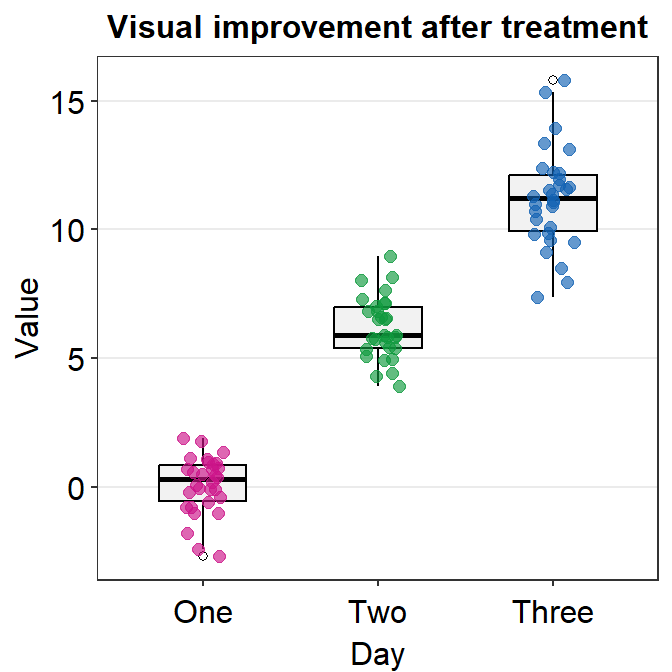• Let’s set the shape to 21.
• Let’s make the border color to white.
• Let’s apply the same color to each Day.
ggplot(data = df, mapping = aes(x = Day, y = Value, group = Subject)) +
sm_slope(labels = c('Day 1','Day 2'), shape = 21, color = 'white', fill = sm_color('blue'))• You could also apply different color for each Day using scale_fill_manual().
ggplot(data = df, mapping = aes(x = Day, y = Value, group = Subject, fill = Day)) +
sm_slope(labels = c('Day 1','Day 2'), shape = 21, color = 'white') +
scale_fill_manual(values = sm_color('blue','orange'))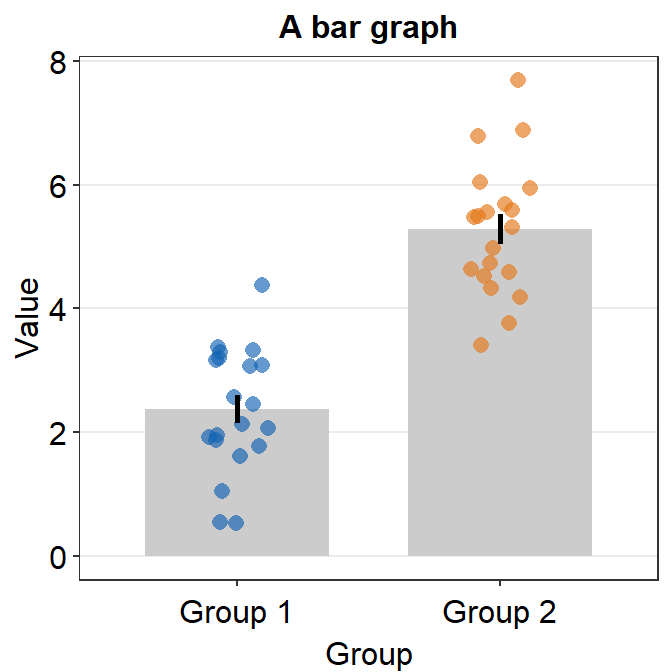• You can also change the line color and other aesthetics. For more information, please type ?sm_slope.
ggplot(data = df, mapping = aes(x = Day, y = Value, group = Subject, fill = Day)) +
sm_slope(labels = c('Day 1','Day 2'), shape = 21, color = 'white',
fill = sm_color('blue'), line_color = '#ADD8E6',
line_size = 0.6)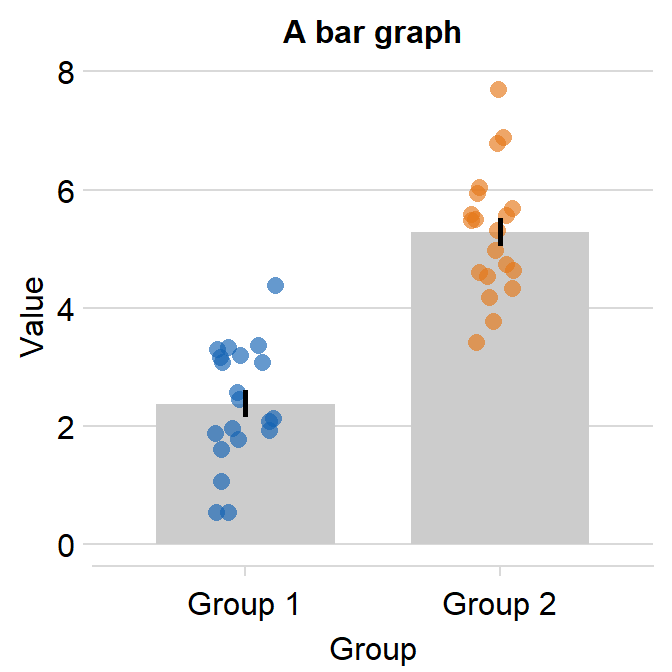## 4.8 A Bland Altman Plot - sm_bland_altman()

• sm_bland_altman() and sm_statBlandAlt() functions can be used to create a Bland-Altman plot.
• The plot is used to measure agreement between two different measurements.
• It is also used to measure test-retest variability of a method.
• Let’s generate random data.
set.seed(1)
first <- rnorm(20)
second <- rnorm(20)
df3 <- as_tibble(cbind(first,second)) # requires library(tidyverse)
• Now let’s draw a Bland Altman plot using sm_bland_altman(), which requires two arguments: first dataset, second dataset. They have to be numerical vectors of equal length.
• This function automatically uses sm_classic() theme.
sm_bland_altman(df3$first, df3$second, color = sm_color('green')) +
scale_y_continuous(limits = c(-4,4))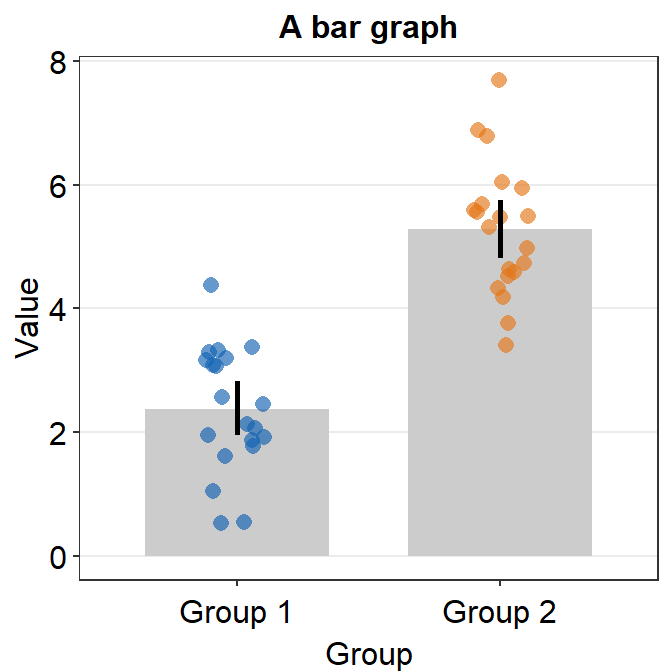• The upper dashed line represents the upper limit of the difference between two measurements (mean difference + 1.96 * standard deviation of the difference).
• The upper dashed line represents the upper limit of the difference between two measurements (mean difference - 1.96 * standard deviation of the difference).
• The middle dashed line represents the mean difference.
• The shaded region is the 95% confidence interval of the difference between the two measuremnts from one-sample t-test (difference vs 0).
• If the shaded region includes 0 in the y-axis, then there is no significant difference (p > 0.05) between 0 and the difference.
• If it does not include 0, then there is a significant difference. This indicates that the two measurement results are considerably different.
• I usually label them with annotate(), which is a function from ggplot2. This process can be tedious.
• Also, sm_statBlandAlt() calculates the statistical values that are necessary to draw a Bland-Altman plot, such as the mean difference, upper and lower limits. This function is used to annotate the values in the plot.
• The arguments for this function are first and second datasets, just like in sm_bland_altman().
res <- sm_statBlandAlt(df3$first,df3$second) # store the results in res variable

sm_bland_altman(df3$first, df3$second, color = sm_color('green')) +
scale_y_continuous(limits = c(-4,4)) +
annotate('text', label = 'Mean', x = -1, y = res$mean_diff + 0.4) + annotate('text', label = signif(res$mean_diff,3), x = -1, y = res$mean_diff - 0.4) + annotate('text', label = 'Upper limit', x = 1.2, y = res$upper_limit + 0.4) +
annotate('text', label = signif(res$upper_limit,3), x = 1.2, y = res$upper_limit - 0.4) +
annotate('text', label = 'Lower limit', x = 1.2, y = res$lower_limit + 0.4) + annotate('text', label = signif(res$lower_limit,3), x = 1.2, y = res$lower_limit-0.4)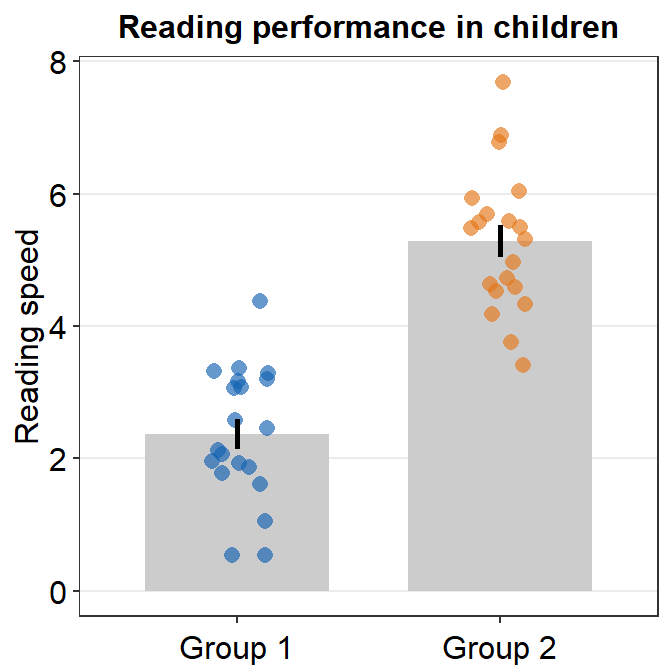• Let’s change the border color of the circles to white. To do so, we will have to change their shape to 21. sm_bland_altman(df3$first, df3$second, shape = 21, fill = sm_color('green'), color = 'white') + scale_y_continuous(limits = c(-4,4)) + annotate('text', label = 'Mean', x = -1, y = res$mean_diff + 0.4) +
annotate('text', label = signif(res$mean_diff,3), x = -1, y = res$mean_diff - 0.4) +
annotate('text', label = 'Upper limit', x = 1.2, y = res$upper_limit + 0.4) + annotate('text', label = signif(res$upper_limit,3), x = 1.2, y = res$upper_limit - 0.4) + annotate('text', label = 'Lower limit', x = 1.2, y = res$lower_limit + 0.4) +
annotate('text', label = signif(res$lower_limit,3), x = 1.2, y = res$lower_limit-0.4)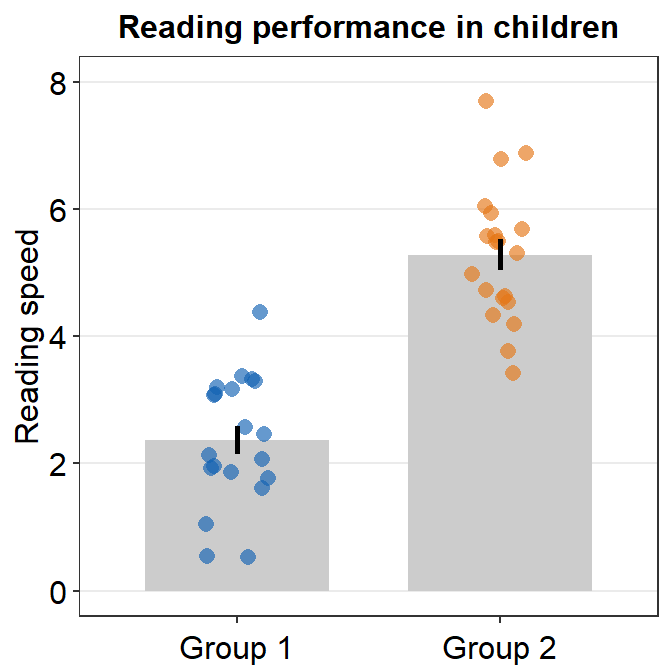## 4.9 Raincloud plot - sm_raincloud()

• sm_raincloud() generates a raincloud plot.
• It also has arguments borders and legends, both of which have been set to FALSE as defaults.
• A raincloud plot is a combination of jittered points, a boxplot and a violin plot.
• However, this plot can be visually crowded. Some people like to use raincloud plots, some do not. So, the choice to use it is entirely yours.
• Let’s generate some random data.
set.seed(2) # generate random data
day1 = rnorm(20,0,1)
day2 = rnorm(20,5,1)
day3 = rnorm(20,6,1.5)
day4 = rnorm(20,7,2)
Subject <- rep(paste0('S',seq(1:20)), 4)
Data <- data.frame(Value = matrix(c(day1,day2,day3,day4),ncol=1))
Day <- rep(c('Day 1', 'Day 2', 'Day 3', 'Day 4'), each = length(day1))
df2 <- cbind(Subject, Data, Day)
• The x-axis variable column has to have the right level. If not, you should convert the column as factor and establish the levels correctly.
• Now let’s draw a raincloud plot using sm_raincloud().
sm_raincloud(data = df2, x = Day, y = Value)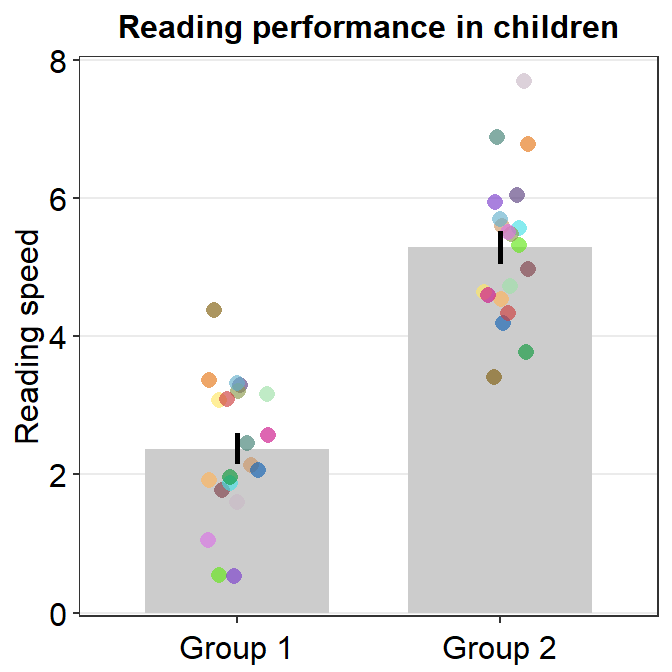• Let’s change the x-axis labels.
sm_raincloud(data = df2, x = Day, y = Value) +
scale_x_continuous(limits = c(0.25,4.75), labels = c('1', '2', '3', '4'), breaks = c(1,2,3,4)) +
xlab('Day')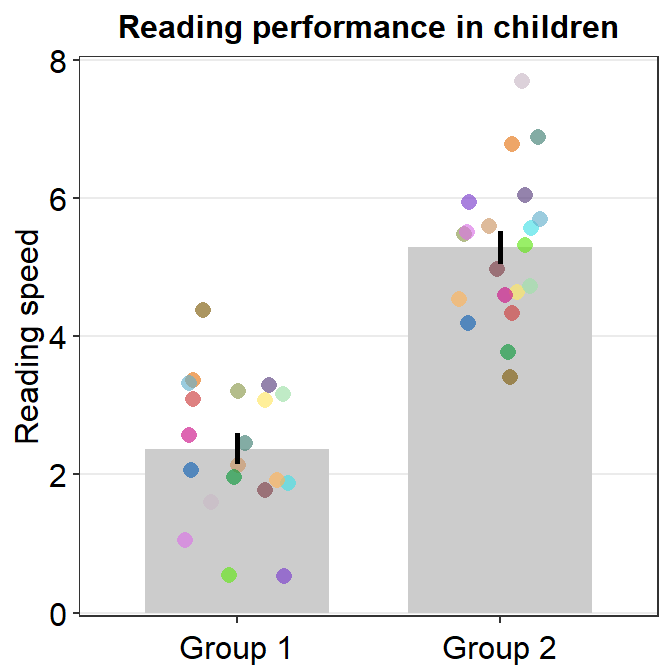• The filling colors of the violin plots and boxplots can be modified by using scale_fill_manual().
• The border color of the violin plot can be changed by using scale_color_manual().
• I will set it transparent to remove the border of the violin plots.
• The color of the points can be used by either of the 2 functions depending on the shape, which can be set within sm_raincloud().
sm_raincloud(data = df2, x = Day, y = Value, boxplot_alpha = 0.5,
color = 'white', shape = 21, sep_level = 2) +
scale_x_continuous(limits = c(0.25,4.75), labels = c('1', '2', '3', '4'), breaks = c(1,2,3,4)) +
xlab('Day') +
scale_color_manual(values = rep('transparent',4)) +
scale_fill_manual(values = sm_palette(4))• sep_level is an argument to specify the degree of separation among points, boxplots and violin plots. When sep_level = 0, they will all be crowded. When sep_level = 4, they will all be separated from each other.
• I personally prefer when the boxplot and violin plots are together, but not the points. So I set the default to sep_level = 2.
• Shown below is an example when sep_level = 4 with a horizontal grid theme sm_hgrid().
sm_raincloud(data = df2, x = Day, y = Value, boxplot_alpha = 0.5,
color = 'white', shape = 21, sep_level = 4) +
scale_x_continuous(limits = c(0.25,4.75), labels = c('1', '2', '3', '4'), breaks = c(1,2,3,4)) +
xlab('Day') +
scale_color_manual(values = rep('transparent',4)) +
scale_fill_manual(values = sm_palette(4)) +
sm_hgrid()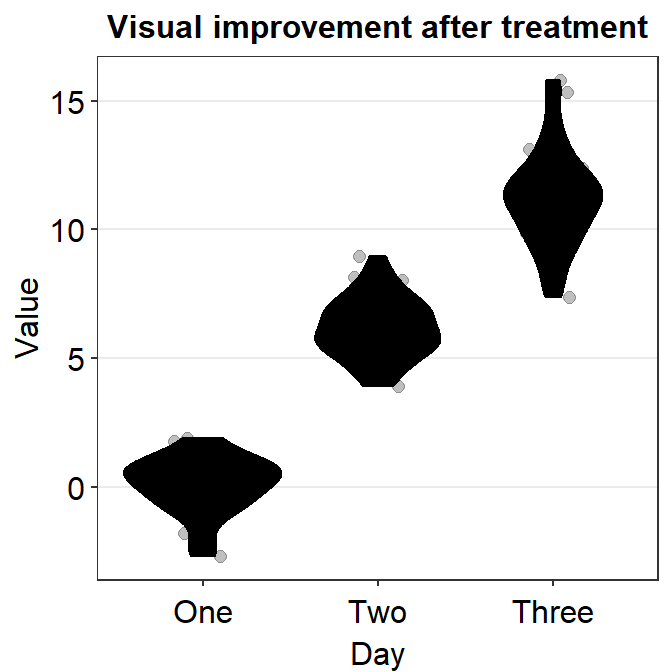• You can also flip the raincloud plot by setting which_side to left.
sm_raincloud(data = df2, x = Day, y = Value, boxplot_alpha = 0.5,
color = 'white', shape = 21, sep_level = 2, which_side = 'left') +
scale_x_continuous(limits = c(0.25,4.75), labels = c('1', '2', '3', '4'), breaks = c(1,2,3,4)) +
xlab('Day') +
scale_color_manual(values = rep('transparent',4)) +
scale_fill_manual(values = sm_palette(4))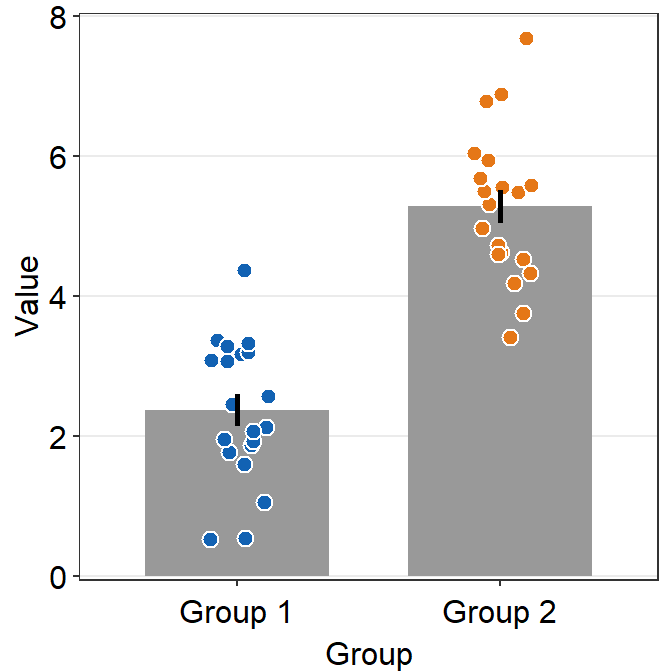• So far the distribution plots (violin plots) have been vertical. We can change their configuration by setting vertical = FALSE.
sm_raincloud(data = df2, x = Day, y = Value, boxplot_alpha = 0.5,
color = 'white', shape = 21, sep_level = 2, which_side = 'left', vertical = FALSE) +
scale_x_continuous(limits = c(0.25,4.75), labels = c('1', '2', '3', '4'), breaks = c(1,2,3,4)) +
xlab('Day') +
scale_color_manual(values = rep('transparent',4)) +
scale_fill_manual(values = sm_palette(4))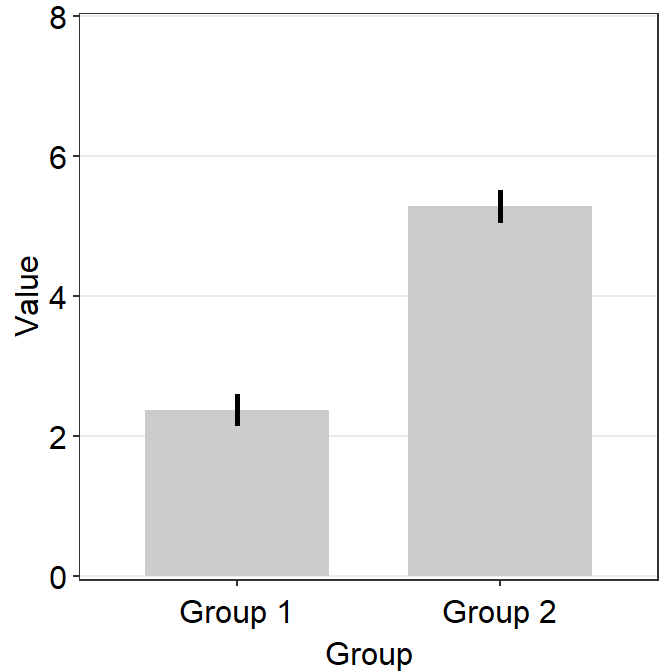• The orientation is not correct, so let’s change it by setting which_side = 'right'.
sm_raincloud(data = df2, x = Day, y = Value, boxplot_alpha = 0.5,
color = 'white', shape = 21, sep_level = 2, which_side = 'right', vertical = FALSE) +
scale_x_continuous(limits = c(0.25,4.75), labels = c('1', '2', '3', '4'), breaks = c(1,2,3,4)) +
xlab('Day') +
scale_color_manual(values = rep('transparent',4)) +
scale_fill_manual(values = sm_palette(4))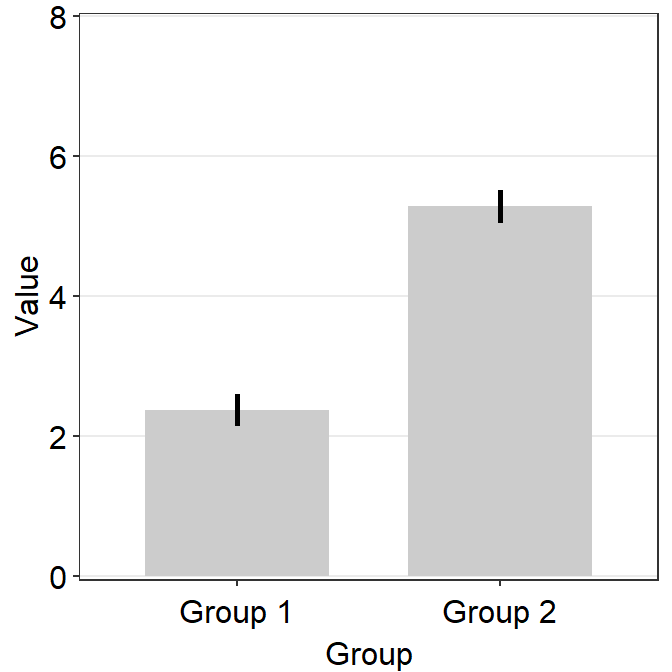## 4.10 Overriding Defaults of smplot’s Themes

• You can override all the defaults by adding theme() object to your ggplot2 graph.

• Here is a bar graph.

ggplot(data = df, mapping = aes(x = Day, y = Value, fill = Day)) +
sm_bar(shape = 21, color = 'white', bar_fill_color = 'gray80') +
scale_fill_manual(values = sm_color('blue','orange'))• Now let’s remove the x-axis title Day.
ggplot(data = df, mapping = aes(x = Day, y = Value, fill = Day)) +
sm_bar(shape = 21, color = 'white', bar_fill_color = 'gray80') +
scale_fill_manual(values = sm_color('blue','orange')) +
theme(axis.title.x = element_blank())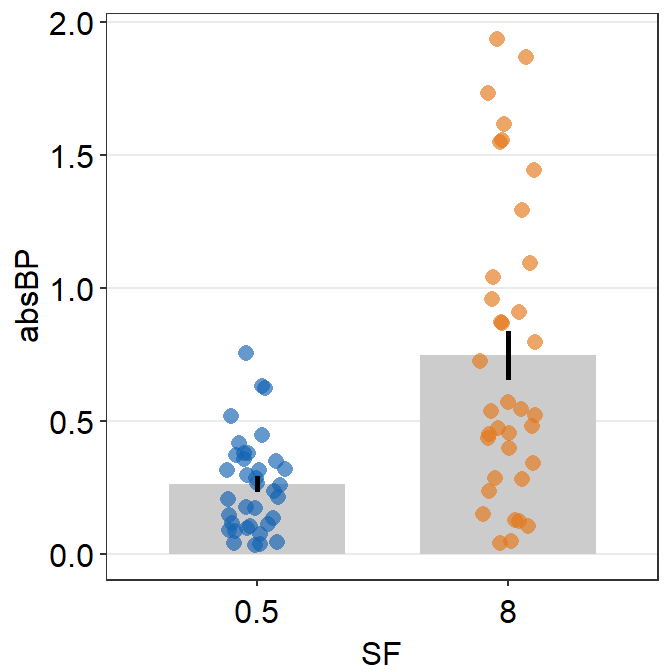• Let’s customise the graph more by changing the y-axis title and adding a main title.
ggplot(data = df, mapping = aes(x = Day, y = Value, fill = Day)) +
sm_bar(shape = 21, color = 'white', bar_fill_color = 'gray80') +
scale_fill_manual(values = sm_color('blue','orange')) +
theme(axis.title.x = element_blank()) +
ylab('Value') +
ggtitle('Reading performance in children')## 4.11 Overriding Defaults of smplot Colors

• sm_color('blue) prints a hex code of the blue. Likewise, sm_color('blue','orange') prints out two hex codes, one for blue and another for orange.

• Therefore, instead of using sm_color() function to call forth the colors, you can directly write the hex codes of colors that are not included in smplot.

my_colors <- c('#ff1493', '#483d8B') # pink and lavender

ggplot(data = df, mapping = aes(x = Day, y = Value, fill = Day)) +
sm_bar(shape = 21, color = 'white', bar_fill_color = 'gray80') +
scale_fill_manual(values = my_colors)